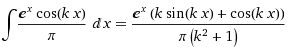# Help finding fourier coefficient

## Homework Statement

Trying to find the Nth degree fourier polynomial of

f(x) =e^x for x between -pi to pi.

## The Attempt at a Solution

I'm trying to calculate ak which is given by

1/∏ ∫e^x cos kx dx from -∏ to ∏

I'm getting stuck here trying to use integration by parts. The integral in integration by parts is basically recursive no matter what I set for u or dv.

jbunniii